#（转）Introductory guide to Generative Adversarial Networks (GANs) and their promise!

0
0
01. 云栖社区>
2. 博客>
3. 正文

## （转）Introductory guide to Generative Adversarial Networks (GANs) and their promise!

wangxiaocvpr 2017-06-19 17:16:00 浏览608

# Introductory guide to Generative Adversarial Networks (GANs) and their promise!

## Introduction

Neural Networks have made great progress. They now recognize images and voice at levels comparable to humans. They are also able to understand natural language with a good accuracy.

But, even then, the talk of automating human tasks with machines looks a bit far fetched. After all, we do much more than just recognizing image / voice or understanding what people around us are saying – don’t we?

Let us see a few examples where we need human creativity (at least as of now):

• Train an artificial author which can write an article and explain data science concepts to a community in a very simplistic manner by learning from past articles on Analytics Vidhya
• You are not able to buy a painting from a famous painter which might be too expensive. Can you create an artificial painter which can paint like any famous artist by learning from his / her past collections?

Do you think, these tasks can be accomplished by machines? Well – the answer might surprise you

These are definitely difficult to automate tasks, but Generative Adversarial Networks (GANs) have started making some of these tasks possible.

If you feel intimidated by the name GAN – don’t worry! You will feel comfortable with them by end of this article.

In this article, I will introduce you to the concept of GANs and explain how they work along with the challenges. I will also let you know of some cool things people have done using GAN and give you links to some of the important resources for getting deeper into these techniques.

## Excuse me, but what is a GAN?

Yann LeCun, a prominent figure in Deep Learning Domain said in his Quora session that

“(GANs), and the variations that are now being proposed is the most interesting idea in the last 10 years in ML, in my opinion.”

Surely he has a point. When I saw the implicationsGenerative Adversarial Networks (GANs) can have if they were executed to their fullest extent, I was impressed too.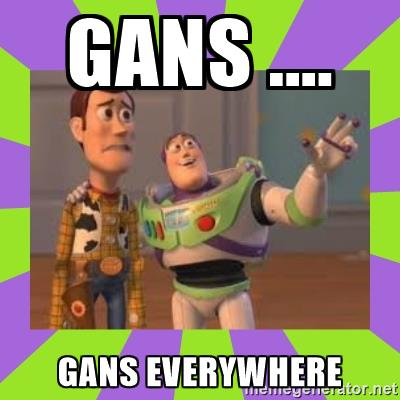But what is a GAN?

Let us take an analogy to explain the concept:

If you want to get better at something, say chess; what would you do? You would compete with an opponent better than you. Then you would analyze what you did wrong, what he / she did right, and think on what could you do to beat him / her in the next game.

You would repeat this step until you defeat the opponent. This concept can be incorporated to build better models. So simply, for getting a powerful hero (viz generator), we need a more powerful opponent (viz discriminator)!

### Another analogy from real life

A slightly more real analogy can be considered as a relation between forger and an investigator.The task of a forger is to create fraudulent imitations of original paintings by famous artists. If this created piece can pass as the original one, the forger gets a lot of money in exchange of the piece.

On the other hand, an art investigator’s task is to catch these forgers who create the fraudulent pieces. How does he do it? He knows what are the properties which sets the original artist apart and what kind of painting he should have created. He evaluates this knowledge with the piece in hand to check if it is real or not.

This contest of forger vs investigator goes on, which ultimately makes world class investigators (and unfortunately world class forger); a battle between good and evil.

## How do GANs work?

We got a high level overview of GANs. Now, we will go on to understand their nitty-gritty of these things.

As we saw, there are two main components of a GAN – Generator Neural Network and Discriminator Neural Network.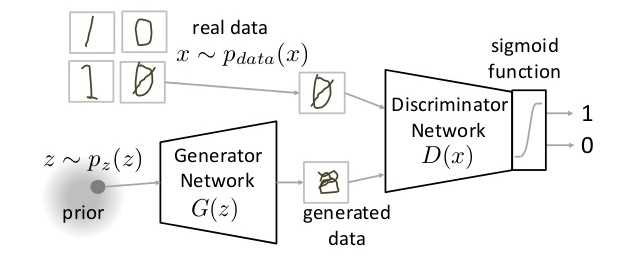The Generator Network takes an random input and tries to generate a sample of data. In the above image, we can see that generator G(z) takes a input z from p(z), where  z is a sample from probability distribution p(z). It then generates a data which is then fed into a discriminator network D(x). The task of Discriminator Network is to take input either from the real data or from the generator and try to predict whether the input is real or generated. It takes an input x from pdata(x) where pdata(x) is our real data distribution. D(x) then solves a binary classification problem using sigmoid function giving output in the range 0 to 1.

Let us define the notations we will be using to formalize our GAN,

Pdata(x) -> the distribution of real data
X -> sample from pdata(x)
P(z) -> distribution of generator
Z -> sample from p(z)
G(z) -> Generator Network
D(x) -> Discriminator Network

Now the training of GAN is done (as we saw above) as a fight between generator and discriminator. This can be represented mathematically as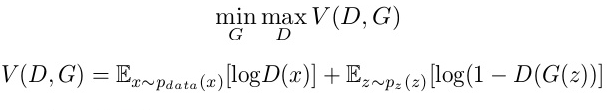In our function V(D, G) the first term is entropy that the data from real distribution (pdata(x)) passes through the discriminator (aka best case scenario). The discriminator tries to maximize this to 1. The second term is entropy that the data from random input (p(z)) passes through the generator, which then generates a fake sample which is then passed through the discriminator to identify the fakeness (aka worst case scenario). In this term, discriminator tries to maximize it to 0 (i.e. the log probability that the data from generated is fake is equal to 0). So overall, the discriminator is trying to maximize our function V.

On the other hand, the task of generator is exactly opposite, i.e. it tries to minimize the function V so that the differentiation between real and fake data is bare minimum. This, in other words is a cat and mouse game between generator and discriminator!

Note: This method of training a GAN is taken from game theory called the minimax game.

### Parts of training GAN

So broadly a training phase has two main subparts and they are done sequentially

• Pass 1: Train discriminator and freeze generator (freezing means setting training as false. The network does only forward pass and no backpropagation is applied)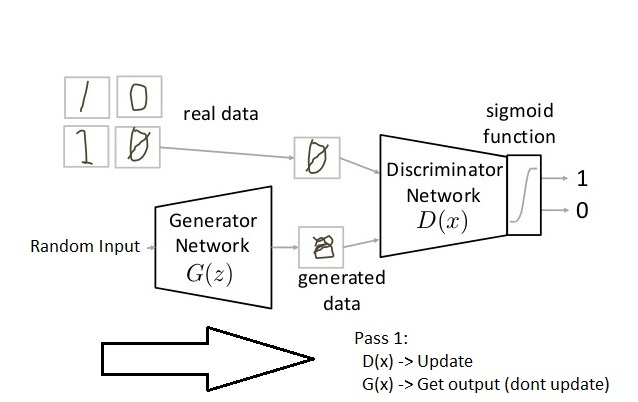• Pass 2: Train generator and freeze discriminator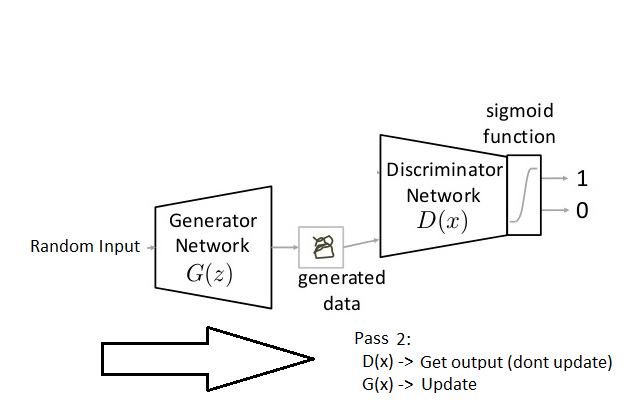### Steps to train a GAN

Step 1: Define the problem. Do you want to generate fake images or fake text. Here you should completely define the problem and collect data for it.

Step 2: Define architecture of GAN. Define how your GAN should look like. Should both your generator and discriminator be multi layer perceptrons, or convolutional neural networks? This step will depend on what problem you are trying to solve.

Step 3: Train Discriminator on real data for n epochs. Get the data you want to generate fake on and train the discriminator to correctly predict them as real. Here value n can be any natural number between 1 and infinity.

Step 4: Generate fake inputs for generator and train discriminator on fake data. Get generated data and let the discriminator correctly predict them as fake.

Step 5: Train generator with the output of discriminator. Now when the discriminator is trained, you can get its predictions and use it as an objective for training the generator. Train the generator to fool the discriminator.

Step 6: Repeat step 3 to step 5 for a few epochs.

Step 7: Check if the fake data manually if it seems legit. If it seems appropriate, stop training, else go to step 3. This is a bit of a manual task, as hand evaluating the data is the best way to check the fakeness. When this step is over, you can evaluate whether the GAN is performing well enough.

Now just take a breath and look at what kind of implications this technique could have. If hypothetically you had a fully functional generator, you can duplicate almost anything. To give you examples, you can generate fake news; create books and novels with unimaginable stories; on call support and much more. You can have artificial intelligence as close to reality; a true artificial  intelligence! That’s the dream!!

## Challenges with GANs

You may ask, if we know what could these beautiful creatures (monsters?) do; why haven’t something happened? This is because we have barely scratched the surface. There’s so many roadblocks into building a “good enough” GAN and we haven’t cleared many of them yet. There’s a whole area of research out there just to find “how to train a GAN

The most important roadblock while training a GAN is stability. If you start to train a GAN, and the discriminator part is much powerful that its generator counterpart, the generator would fail to train effectively. This will in turn affect training of your GAN. On the other hand, if the discriminator is too lenient; it would let literally any image be generated. And this will mean that your GAN is useless.

Another way to glance at stability of GAN is to look as a holistic convergence problem. Both generator and discriminator are fighting against each other to get one step ahead of the other. Also, they are dependent on each other for efficient training. If one of them fails, the whole system fails. So you have to make sure they don’t explode.

This is kind of like the shadow in Prince of Persia game . You have to defend yourself from the shadow, which tries to kill you. If you kill the shadow you die, but if you don’t do anything, you will definitely die!

There are other problems too, which I will list down here. (Reference: http://www.iangoodfellow.com/slides/2016-12-04-NIPS.pdf)

Note: Below mentioned images are generated by a GAN trained on ImageNet dataset.

• Problem with Counting: GANs fail to differentiate how many of a particular object should occur at a location. As we can see below, it gives more number of eyes in the head than naturally present.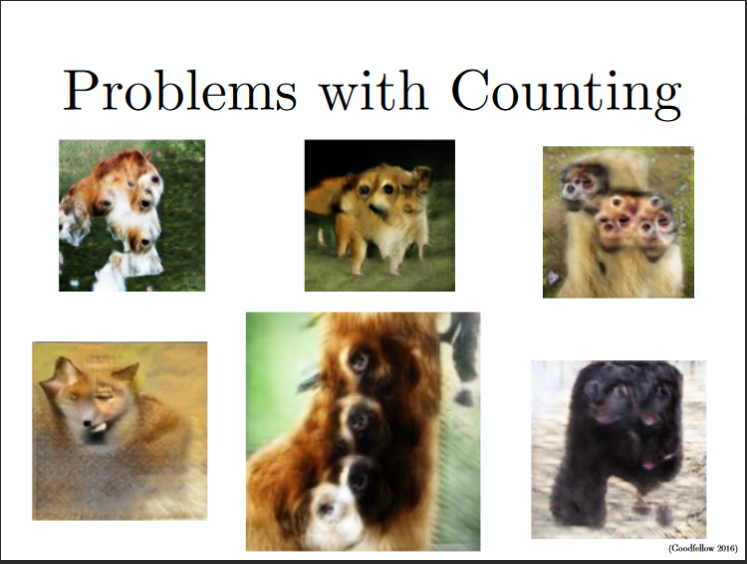• Problems with Perspective: GANs fail to adapt to 3D objects. It doesn’t understand perspective, i.e.difference between frontview and backview. As we can see below, it gives flat (2D) representation of 3D objects.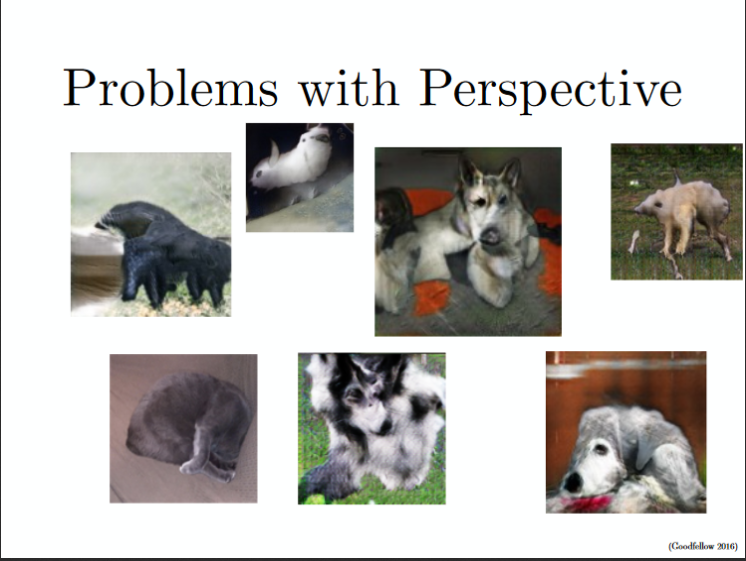• Problems with Global Structures: Same as the problem with perspective, GANs do not understand a holistic structure. For example, in the bottom left image, it gives a generated image of a quadruple cow, i.e. a cow standing on its hind legs and simultaneously on all four legs. That is definitely not possible in real life!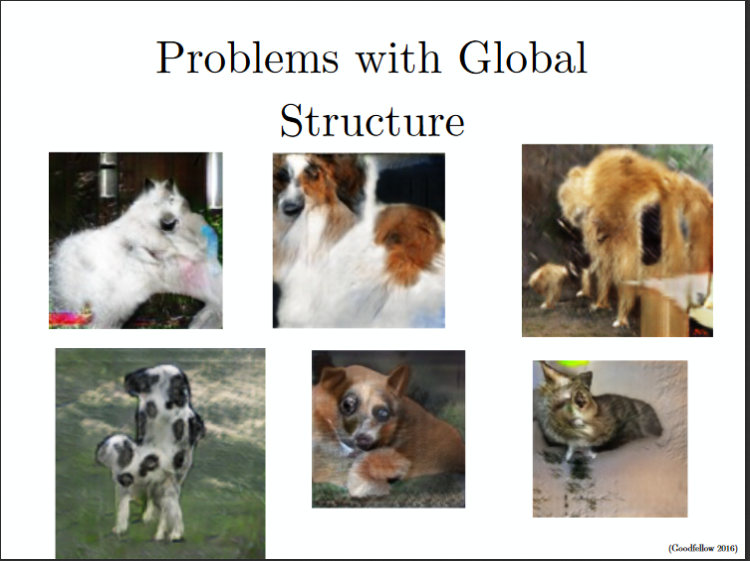A substantial research is being done to take care of these problems. Newer types of models are proposed which give more accurate results than previous techniques, such as DCGAN, WassersteinGAN etc

## Implementing a Toy GAN

Lets see a toy implementation of GAN to strengthen our theory. We will try to generate digits by training a GAN on Identify the Digits dataset. A bit about the dataset; the dataset contains 28×28 images which are black and white. All the images are in “.png” format. For our task, we will only work on the training set. You can download the dataset from here.

You also need to setup the libraries , namely

Before starting with the code, let us understand the internal working thorugh pseudocode. A pseudocode of GAN training can be thought out as follows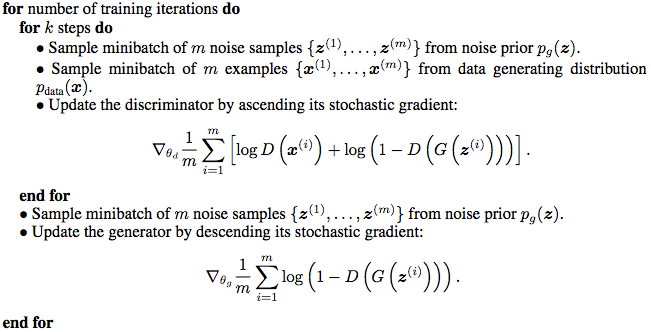Note: This is the first implementation of GAN that was published in the paper. Numerous improvements/updates in the pseudocode can be seen in the recent papers such as adding batch normalization in the generator and discrimination network, training generator k times etc.

Let us first import all the modules

```# import modules
%pylab inline

import os
import numpy as np
import pandas as pd

import keras
from keras.models import Sequential
from keras.layers import Dense, Flatten, Reshape, InputLayer
from keras.regularizers import L1L2```

To have a deterministic randomness, we set a seed value

```# to stop potential randomness
seed = 128
rng = np.random.RandomState(seed)```

We set the path of our data and working directory

```# set path
root_dir = os.path.abspath('.')
data_dir = os.path.join(root_dir, 'Data')```

```# load data

temp = []
for img_name in train.filename:
image_path = os.path.join(data_dir, 'Train', 'Images', 'train', img_name)
img = img.astype('float32')
temp.append(img)

train_x = np.stack(temp)

train_x = train_x / 255.```

To visualize what our data looks like, let us plot one of the image

```# print image
img_name = rng.choice(train.filename)
filepath = os.path.join(data_dir, 'Train', 'Images', 'train', img_name)

pylab.imshow(img, cmap='gray')
pylab.axis('off')
pylab.show()```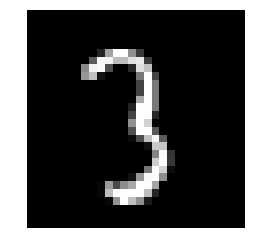Define variables which we will be using later

`# define variables`

# define vars g_input_shape = 100 d_input_shape = (28, 28) hidden_1_num_units = 500 hidden_2_num_units = 500 g_output_num_units = 784 d_output_num_units = 1 epochs = 25 batch_size = 128

Now define our generator and discriminator networks

```# generator
model_1 = Sequential([
Dense(units=hidden_1_num_units, input_dim=g_input_shape, activation='relu', kernel_regularizer=L1L2(1e-5, 1e-5)),

Dense(units=hidden_2_num_units, activation='relu', kernel_regularizer=L1L2(1e-5, 1e-5)),

Dense(units=g_output_num_units, activation='sigmoid', kernel_regularizer=L1L2(1e-5, 1e-5)),

Reshape(d_input_shape),
])

# discriminator
model_2 = Sequential([
InputLayer(input_shape=d_input_shape),

Flatten(),

Dense(units=hidden_1_num_units, activation='relu', kernel_regularizer=L1L2(1e-5, 1e-5)),

Dense(units=hidden_2_num_units, activation='relu', kernel_regularizer=L1L2(1e-5, 1e-5)),

Dense(units=d_output_num_units, activation='sigmoid', kernel_regularizer=L1L2(1e-5, 1e-5)),
])```

Here is the architecture of our networks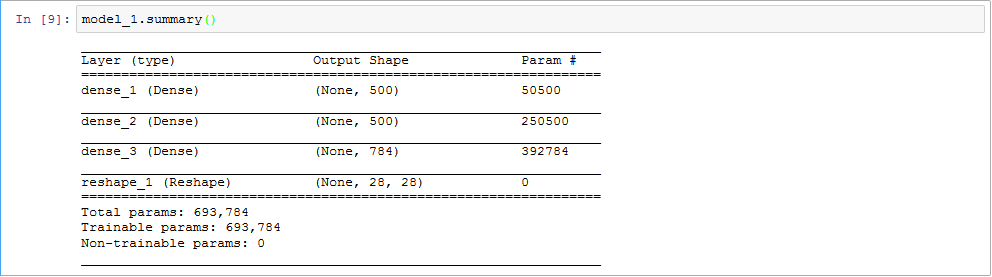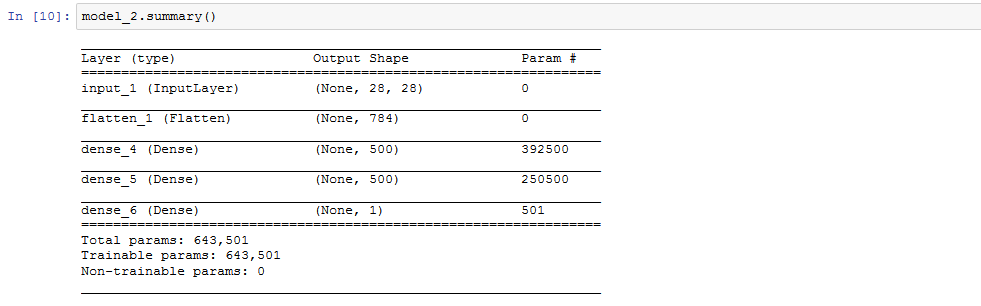We will then define our GAN, for that we will first import a few important modules

```from keras_adversarial import AdversarialModel, simple_gan, gan_targets

Let us compile our GAN and start the training

```gan = simple_gan(model_1, model_2, normal_latent_sampling((100,)))

history = model.fit(x=train_x, y=gan_targets(train_x.shape), epochs=10, batch_size=batch_size)```

Here’s how our GAN would look like,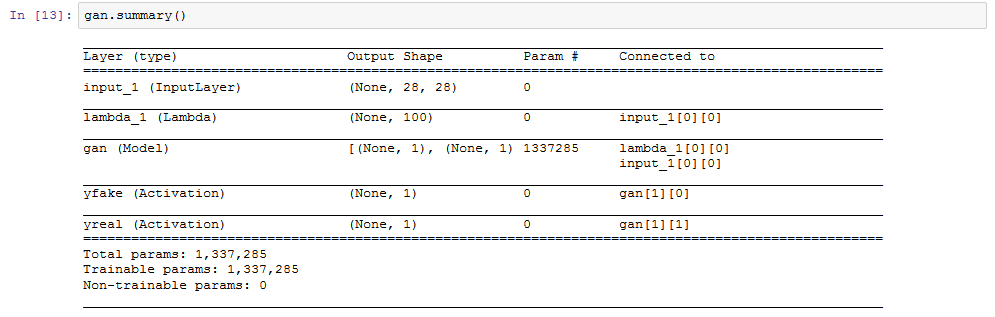We get a graph like after training for 10 epochs.

```plt.plot(history.history['player_0_loss'])
plt.plot(history.history['player_1_loss'])
plt.plot(history.history['loss'])```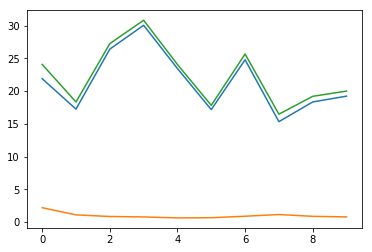After training for 100 epochs, I got the following generated images

```zsamples = np.random.normal(size=(10, 100))
pred = model_1.predict(zsamples)
for i in range(pred.shape):
plt.imshow(pred[i, :], cmap='gray')
plt.show()```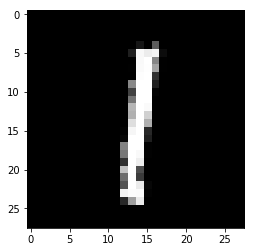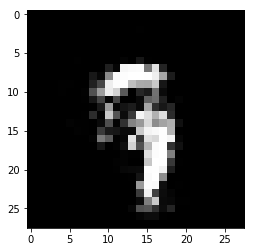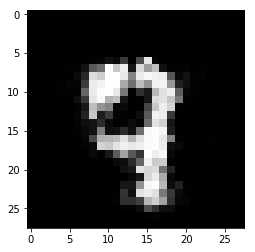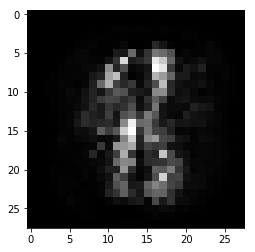And voila! You have built your first generative model!

## Applications of GAN

We saw an overview of how these things work and got to know the challenges of training them. We will now see the cutting edge research that has been done using GANs

• Predicting the next frame in a video : You train a GAN on video sequences and let it predict what would occur next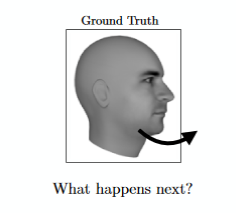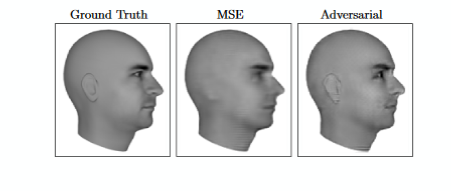Paper : https://arxiv.org/pdf/1511.06380.pdf

• Increasing Resolution of an image : Generate a high resolution photo from a comparatively low resolution.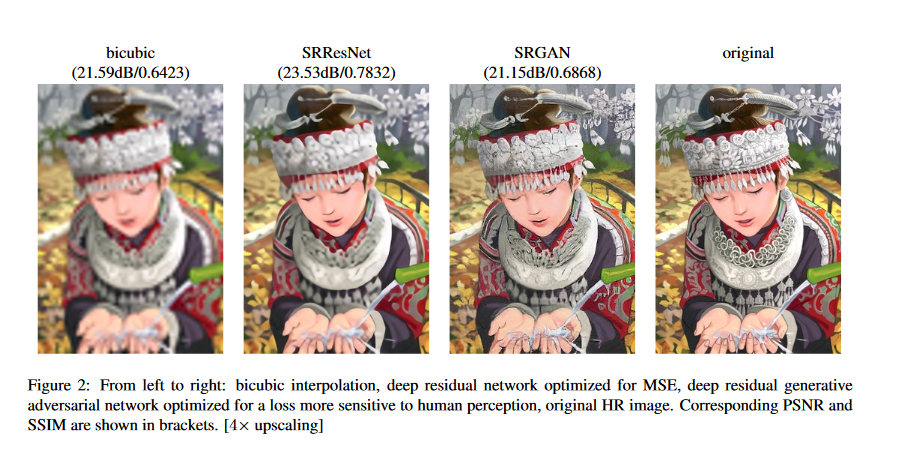Paper: https://arxiv.org/pdf/1609.04802.pdf

• Interactive Image Generation : Draw simple strokes and let the GAN draw an impressive picture for you!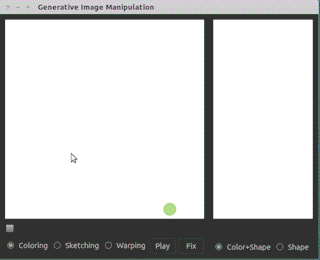• Image to Image Translation : Generate an image from another image. For example, given on the left, you have labels of a street scene and you can generate a real looking photo with GAN. On the right, you give a simple drawing of a handbag and you get a real looking drawing of a handbag.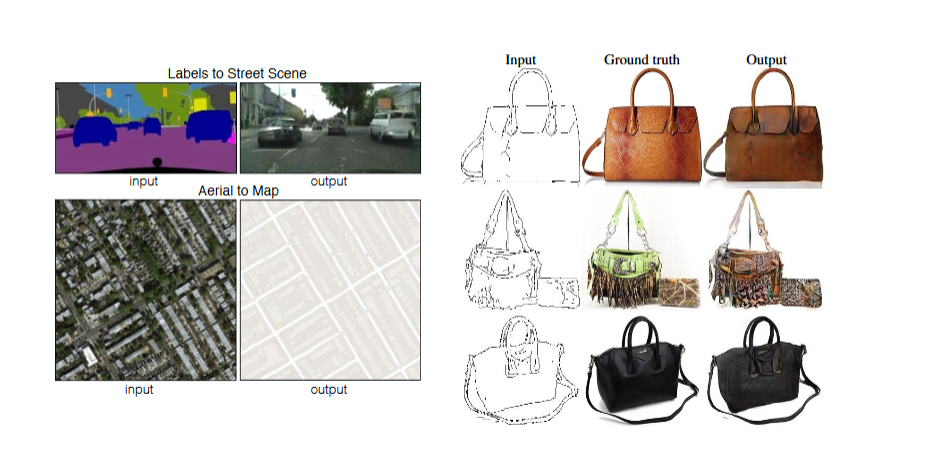Paper: https://arxiv.org/pdf/1611.07004.pdf

• Text to Image Generation : Just say to your GAN what you want to see and get a realistic photo of the target.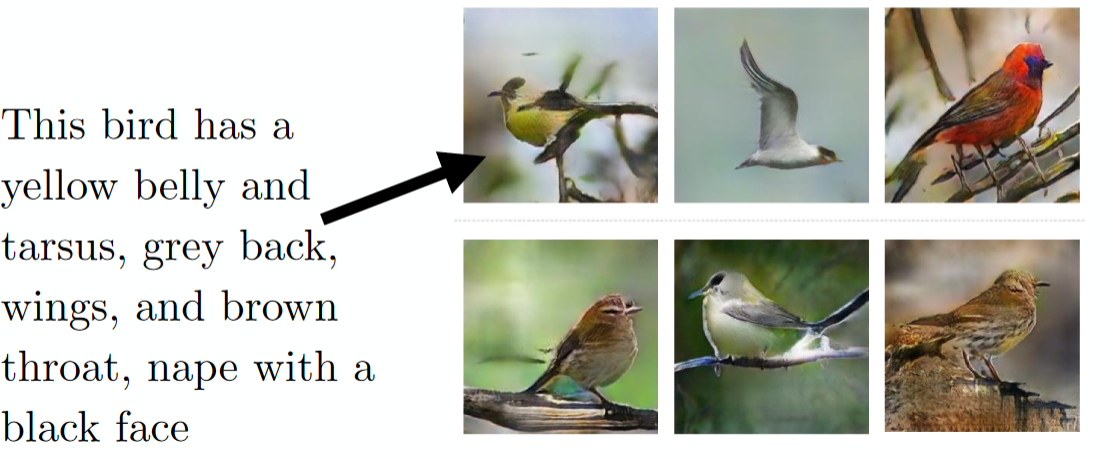Paper : https://arxiv.org/pdf/1605.05396.pdf

## Resources

Here are some resources which you might find helpful to get more in-depth on GAN

## End Notes

Phew! I hope you are now as excited about the future as I was when I first read about GANs. They are set to change what machines can do for us. Think of it – from preparing new recipes of food to creating drawings. The possibilities are endless.

In this article, I tried to cover a general overview of GAN and its applications. GAN is very exciting area and that’s why researchers are so excited about building generative models and you can see that new papers on GANs are coming out more frequently.

If you have any questions on GANs, please feel free to share them with me through comments.

wangxiaocvpr
+ 关注